## A system has two components: A and B. The operating times until failure of the two components are independent and exponentially distributed

Question

A system has two components: A and B. The operating times until failure of the two components are independent and exponentially distributed random variables with parameter 2 for component A, and parameter 3 for component B. The system fails at the first component failure.
(a) – Read section 1.5.2 in the textbook.
(b) – What is the mean time to failure for component A and for component B

in progress 0
6 months 2021-07-20T21:19:17+00:00 1 Answers 23 views 0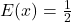— Component A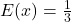— Component B

Step-by-step explanation:

Given

Distribution = Exponential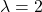— Component A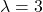— Component B

Solving (a): The mean time of A

The mean of an exponential distribution is: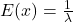We have:— Component ASolving (b): The mean time of B

The mean of an exponential distribution is:We have:— Component B